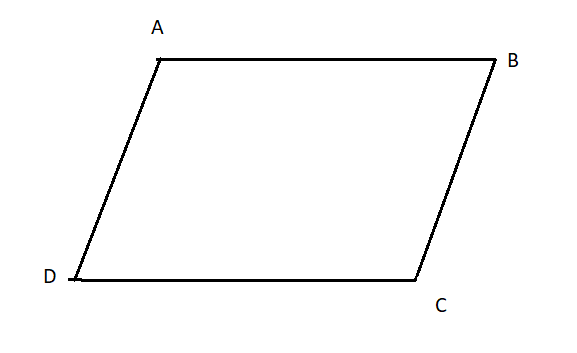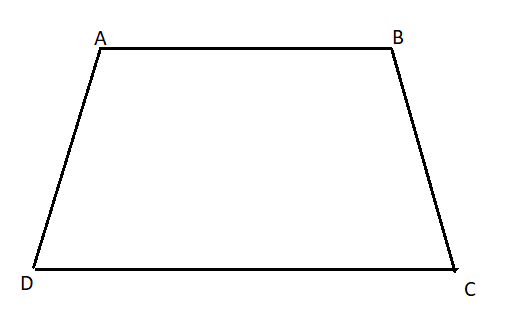QuestionAnswers

# State true or false.Every parallelogram is a trapezium.${\text{A}}{\text{. True}} \\ {\text{B}}{\text{. False}} \\$Hint:-In this question first we need to analyse the parallelogram and its properties. Then analyse the trapezium and its properties and finally check that every property of parallelogram is also the property of trapezium or not.

We know, a parallelogram is a special type of quadrilateral that has equal and parallel opposite sides.
The given figure shows a parallelogram ABCD which has AB parallel to CD and AD parallel to BC.
And AB=CDAnd the trapezium is a quadrilateral, which is defined as a shape with four sides and one set of parallel sides.
The given figure shows a trapezium ABCD which has AB side parallel to CD and two other sides AD and BC which are non-parallel.
$\Rightarrow {\text{AB = CD}} \\ {\text{and AD = BC}} \\$From the above discussion, we observe that the pair of opposite sides of a parallelogram are equal and parallel but in the case of trapezium, this is not true in that only one pair of opposite sides are equal.
Therefore every parallelogram is not a trapezium.
Hence, option B. is correct.

Note:- Whenever you get this type of question the key concept to solve this is to learn about different shapes like quadrilateral, rectangle, square, parallelogram, trapezium, rhombus and their properties.
Where There is a Will There is a Way EssayParallelogram FormulaThe Difference Between an Animal that is A Regulator and One that is A ConformerDiagonal of Parallelogram FormulaLaughter is a Best Medicine EssayCBSE Class 10 Maths Chapter 10 - Circles FormulaTo Find the Weight of a Given Body Using Parallelogram Law of VectorsThe Solid StateTrapeziumImportant Questions for CBSE Class 10 Maths Chapter 10 - CirclesImportant Questions for CBSE Class 6 English A Pact with The Sun Chapter 10 - A Strange Wrestling MatchImportant Questions for CBSE Class 12 Chemistry Chapter 1 - The Solid StateImportant Questions for CBSE Class 10 Maths & Science with AnswersImportant Questions for CBSE Class 10 Maths Chapter 2 - PolynomialsImportant Questions for CBSE Class 10 Maths Chapter 11 - ConstructionsImportant Questions for CBSE Class 10 Maths Chapter 6 - TrianglesImportant Questions for CBSE Class 10 Maths Chapter 15 - ProbabilityImportant Questions for CBSE Class 9 Maths Chapter 10 - CirclesImportant Questions for CBSE Class 6 Maths Chapter 10 - MensurationCBSE Class 10 Hindi A Question Paper 2020Hindi A Class 10 CBSE Question Paper 2009Hindi A Class 10 CBSE Question Paper 2015Hindi A Class 10 CBSE Question Paper 2016Hindi A Class 10 CBSE Question Paper 2012Hindi A Class 10 CBSE Question Paper 2010Hindi A Class 10 CBSE Question Paper 2008Hindi A Class 10 CBSE Question Paper 2014Hindi A Class 10 CBSE Question Paper 2007Hindi A Class 10 CBSE Question Paper 2013NCERT Solutions for Class 12 English Kaliedoscope Poetry Chapter 4 Kubla Khan or a Vision in a Dream a FragmentNCERT Solutions for Class 9 English Moments Chapter 8 - A House is Not a HomeRS Aggarwal Class 8 Mathematics Solutions for Chapter-18 Area of a Trapezium and a PolygonNCERT Solutions for Class 9 Maths Chapter 8 QuadrilateralsRD Sharma Class 12 Maths Solutions Chapter 24 - Scalar Or Dot ProductNCERT Solutions Class 11 English Woven Words Essay Chapter 5 What is a Good BookNCERT Solutions for Class 8 Maths Chapter 3 Understanding QuadrilateralsRS Aggarwal Solutions Class 8 Chapter-18 Area of a Trapezium and a Polygon (Ex 18C) Exercise 18.3RD Sharma Class 12 Maths Solutions Chapter 25 - Vector or Cross ProductRS Aggarwal Solutions Class 8 Chapter-18 Area of a Trapezium and a Polygon (Ex 18B) Exercise 18.2